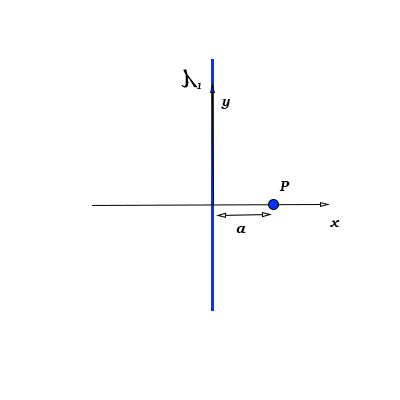# An infinite line of charge with charge density \lambda_1 = 4.6 \mu C/cm is aligned with the...

## Question:

An infinite line of charge with charge density {eq}\lambda_1 = 4.6 \mu C/cm {/eq} is aligned with the {eq}y {/eq}-axis as shown.

What is {eq}E_x(P) {/eq}, the value of the {eq}x {/eq}-component of the electric field produced by by the line of charge at point {eq}P {/eq} which is located at {eq}(x,y)= (a,0) {/eq}, where a {eq}6 cm {/eq}?## Electric Field from Charge Distributions:

The electric field due to a point charge q can be obtained using Coulomb's Law: {eq}\vec E = \dfrac {kq}{r^2} \hat r {/eq}, where k is the Coulomb's constant and r is the distance to the point of observation. The electric field due to a system of charges can be found using superposition principle: the total electric field is the sum of individual electric fields due to each point charge in the system.

Due to symmetry, the only component of electric field at the point P is the x-component; For this component, we can write:

{eq}E_x (P) = \displaystyle \int \limits_{-\infty}^{\infty} \dfrac {k\lambda_1 dy}{y^2 + a^2} \cdot \cos \theta = \int \limits_{-\infty}^{\infty} \dfrac {k\lambda_1 dy}{y^2 + a^2} \cdot \dfrac {a}{\sqrt{y^2 + a^2}} {/eq}

Making the substitution {eq}y = a \sinh x {/eq}, we obtain the following expression:

{eq}E_x (P) = \displaystyle \dfrac {k\lambda_1}{a} \int \limits_{-\infty}^{\infty} \dfrac {dx}{\cosh^2 x} {/eq}

Taking the integral, we obtain:

{eq}E_x (P) = \displaystyle \dfrac {k\lambda_1}{a} (\tanh x) \large|_{-\infty}^{\infty} {/eq}

Using the definition of hyperbolic tangent, we finally obtain:

{eq}E_x (P) = \displaystyle \dfrac {2k\lambda_1}{a} {/eq}

Calculation yields:

{eq}E_x (P) = \displaystyle \dfrac {2\cdot 9\times 10^9 \ N/(m^2 \cdot C^2) \cdot 4.6\times 10^{-4} \ C/ m}{0.06 \ m} = 1.38\times 10^8 \ N/C {/eq}